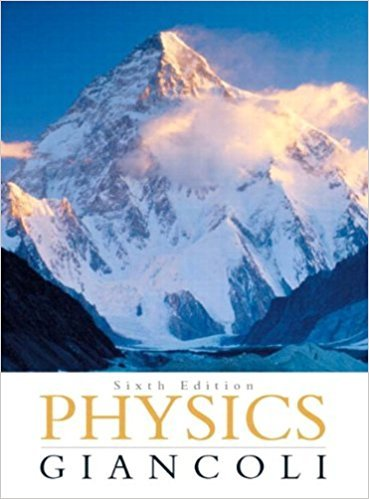×
×

# How long would a day be if the Earth were | Ch 5 - 77GPISBN: 9780321569837 99

## Solution for problem 77GP Chapter 5

Physics: Principles with Applications | 6th Edition

• Textbook Solutions
• 2901 Step-by-step solutions solved by professors and subject experts
• Get 24/7 help from StudySoup virtual teaching assistantsPhysics: Principles with Applications | 6th Edition

4 5 1 343 Reviews
27
5
Problem 77GP

How long would a day be if the Earth were rotating so fast that objects at the equator were apparently weightless?

Step-by-Step Solution:

Solution 77 GP

Step 1 of 3</p>

To find the time taken in a day, if the earth is rotating so fast, that the objects at the equator are apparently weightless.

Step 2 of 3</p>

Let us consider the radial acceleration is equal to acceleration due to gravity ‘g’,….(1)

And let,….(2)

On equating, equations (1) and (2) we get,On solving for ‘T’ we get,….(3)

Step 3 of 3

##### ISBN: 9780321569837

The answer to “How long would a day be if the Earth were rotating so fast that objects at the equator were apparently weightless?” is broken down into a number of easy to follow steps, and 21 words. Physics: Principles with Applications was written by and is associated to the ISBN: 9780321569837. Since the solution to 77GP from 5 chapter was answered, more than 230 students have viewed the full step-by-step answer. This textbook survival guide was created for the textbook: Physics: Principles with Applications, edition: 6. The full step-by-step solution to problem: 77GP from chapter: 5 was answered by , our top Physics solution expert on 09/09/17, 04:43AM. This full solution covers the following key subjects: were, long, EARTH, equator, fast. This expansive textbook survival guide covers 33 chapters, and 4141 solutions.

Unlock Textbook Solution